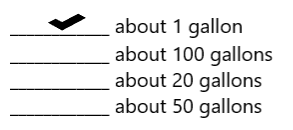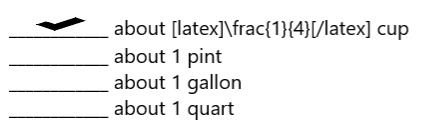# McGraw Hill Math Grade 4 Chapter 11 Lesson 4 Answer Key Customary Units of Capacity

Practice the questions of McGraw Hill Math Grade 4 Answer Key PDF Chapter 11 Lesson 4 Customary Units of Capacity to secure good marks & knowledge in the exams.

## McGraw-Hill Math Grade 4 Answer Key Chapter 11 Lesson 4 Customary Units of Capacity

Solve

Place a check mark next to the best answer.

Question 1.
What is the capacity of a bucket?Explanation:
The capacity of a bucket is about 1 gallon.

Question 2.
What is the capacity of a small bowl?____________ about $$\frac{1}{4}$$ cupExplanation:
The capacity of a small bowl is about $$\frac{1}{4}$$ cup.

Multiply to find each missing number.

Question 3.
3 gal = ____________ pt
24 pt

Explanation:
1 gal = 8 pt
3 gal = 3 x 8 = 24 pt
So, 8 gal = 24 pt.

Question 4.
6 pt = ____________ c
12 c

Explanation:
1 pt = 2 c
6 pt = 6 x 2 = 12 c
So, 6 pt = 12 c.

Question 5.
9 gal = ____________ qt
36 qt

Explanation:
1 gal = 4 qt
9 gal = 9 x 4 = 36 qt
So, 9 gal = 36 qt.

Question 6.
17 gal = ____________ c
272 c

Explanation:
1 gal = 16 c
17 gal = 17 x 16 = 272 c
So, 17 gal = 272 c.

Question 7.
104 qt = ____________ pt
208 pt

Explanation:
1 qt = 2 pt
104 qt = 104 x 2 = 208 pt
So, 104 qt = 208 pt.

Question 8.
365 gal = ____________ c